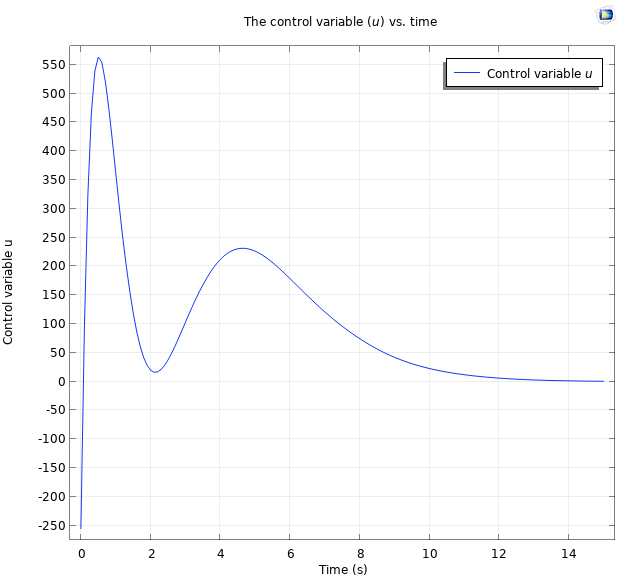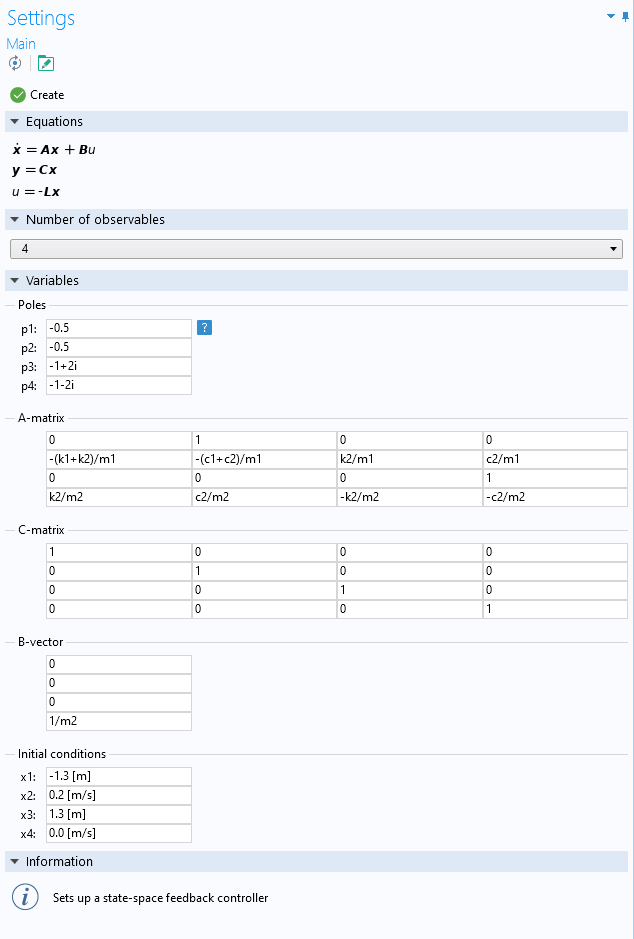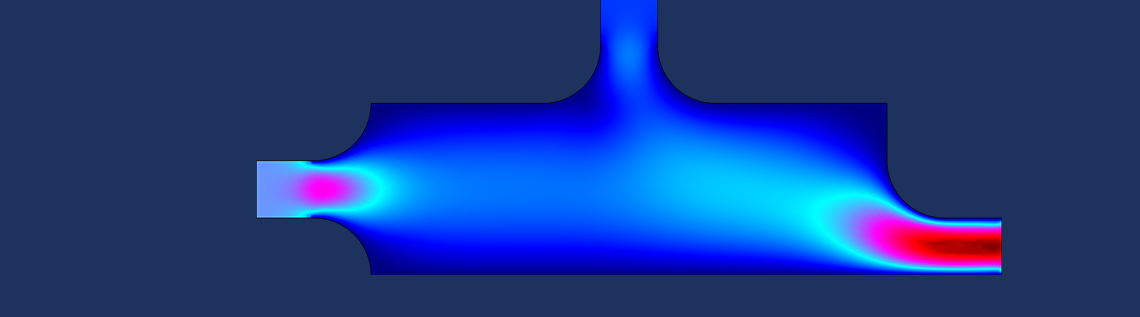# 在控制系统模型中添加一个全状态空间反馈控制器

2021年 3月 23日

### 关于全状态反馈

\dot{\boldsymbol{x}} = \boldsymbol{A}\boldsymbol{x}+\boldsymbol{B}u\,,
\boldsymbol{y}= \boldsymbol{C}\boldsymbol{x} + \boldsymbol{D}u.

### 使用插件实现状态空间反馈控制器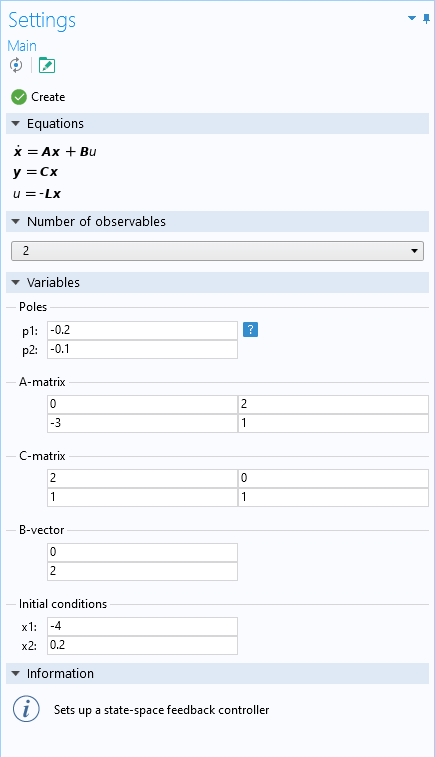### 力对双质量-阻尼-弹簧的示例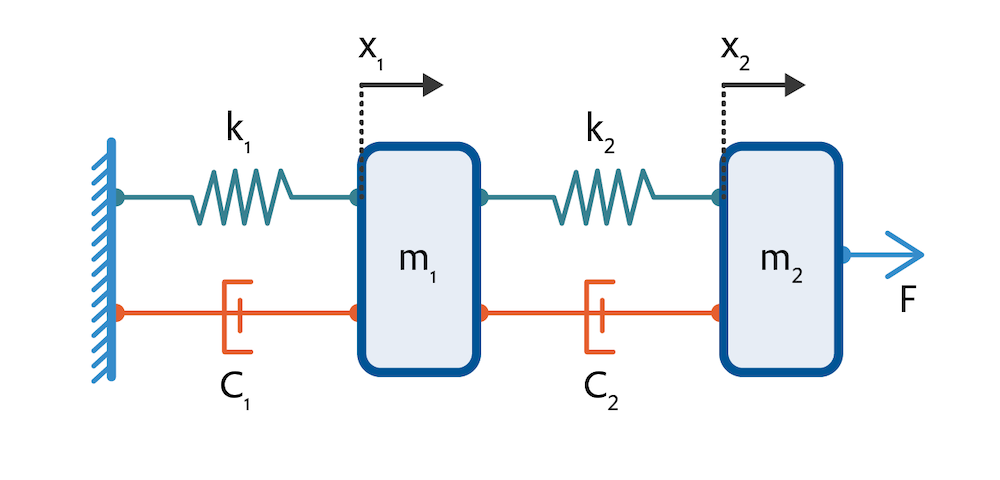m_{1}\ddot{\tilde{x}}_{1} = k_{2}(\tilde{x}_{2}-\tilde{x}_{1})+c_{2}(\dot{\tilde{x}}_{2}-\dot{\tilde{x}}_{1}) – k_{1}\tilde{x}_{1} – c_{1}\dot{\tilde{x}}_{1},
m_{2}\ddot{\tilde{x}}_{2} = F – k_{2}(\tilde{x}_{2}-\tilde{x}_{1})-c_{2}(\dot{\tilde{x}}_{2}-\dot{\tilde{x}}{1}),

\dot{\boldsymbol{x}} = \boldsymbol{A}\boldsymbol{x}+\boldsymbol{B}u\,
\boldsymbol{y}= \boldsymbol{C}\boldsymbol{x},

\boldsymbol{A} = \begin{pmatrix}
0 & 1 & 0 & 0\\
-(k_{1}+k_{2})/m_{1}& -(c_{1}+c_{2})/m_{1} & k_{2}/m_{1} & c_{2}/m_{1}\\
0 & 0 & 0 & 1\\
k_{2}/m_{2} & c_{2}/m_{2} & -k_{2}/m_{2} & -c_{2}/m_{2}
\end{pmatrix} \text{ 和 } \boldsymbol{B} = \begin{pmatrix}
0 \\
0\\
0\\
1/m_{2}
\end{pmatrix}.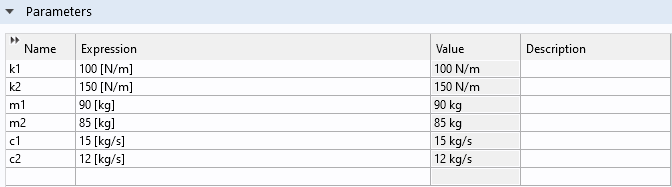25 s 内两个质量点的位置，此时所有极点都位于 -0.5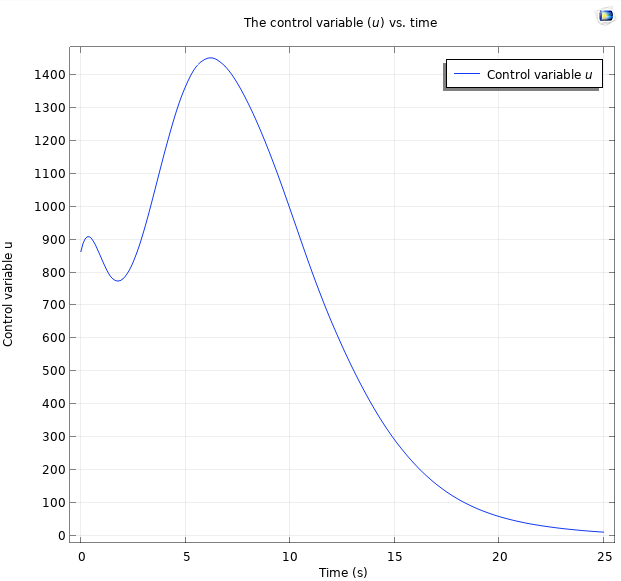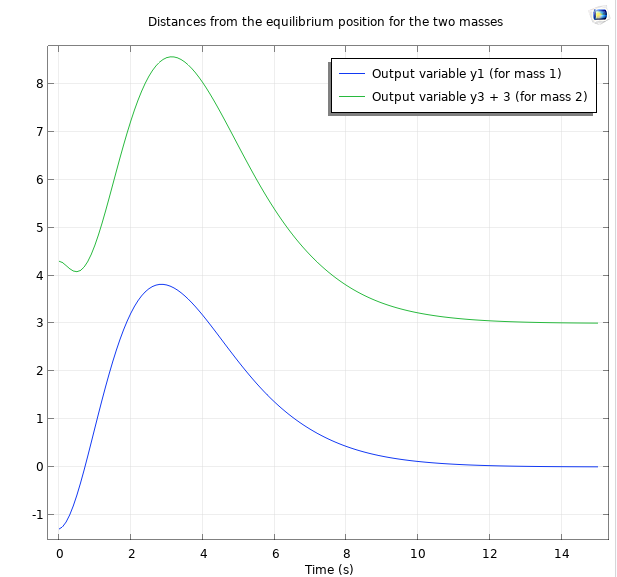15s 的位置图，此时所有极点都位于 -1